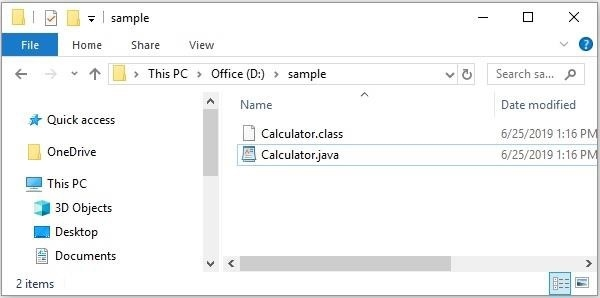# What is the reason behind the error “Could not found or load main class” in java?

When write a Java program/class first of all you need to compile it using the javac command as −

javac [name of the class].java

If your program gets compiled without errors, a .class file (byte code) is created with the specified name. Then you need to execute it using the java command (JVM) as −

java [class name]

## Example

Suppose we Have created a simple class Calculator which adds two or, three numbers in the file with name Calculator.java in the path D:\sample as −

public class Calculator {
int addition(int a , int b){
int result = a+b;
return result;
}
int addition(int a , int b, int c){
int result = a+b+c;
return result;
}
public static void main(String args[]){
Calculator obj = new Calculator();
}
}

## Compilation

First of all, we should compile the Calculator.java using the javac command as −

D:\sample>javac Calculator.java

If this program executes without compilation errors a .class file with name Calculator.class will be generated in the current folder as −## Execution

Then, we can execute the generated byte code using the java command (JVM) as −

java Calculator

## Output

25
40

While executing, when JVM does not find a .class file with the specified name then a run time error occurs saying “Could not found or load main class” error as −

D:\sample>java Calculator
Error: Could not find or load main class Calculator
Caused by: java.lang.ClassNotFoundException: Calculator

To avoid this error, you need to specify the absolute (including packages) name of the .class file (just name) which is in the current directory.

If you see this error while executing a program −

• Wrong class name − You might have specified the wrong class name.
• Wrong case − You need to specify the name of the class with same case Example.java is different from example.java.
• Wrong package − You might have created the .class file in a package and tried to execute without package name or with wrong package name.

## Example

package sample.example;
class Calculator {
int addition(int a , int b){
int result = a+b;
return result;
}
public static void main(String args[]){
Calculator obj = new Calculator();
}
}

Here we have specified a package name in the program and compiled it as −

D:\sample>javac -d . Calculator.java

While execute we need to specify the correct package name in which the .class file exists as −

D:\sample>java sample.example.Calculator
25
• Inclusion of .class extension − While executing a file there is no need to include the .class extension in your program you just need to specify the name of the class file.
D:\sample>java Calculator.class
Error: Could not find or load main class Calculator.class
Caused by: java.lang.ClassNotFoundException: Calculator.class